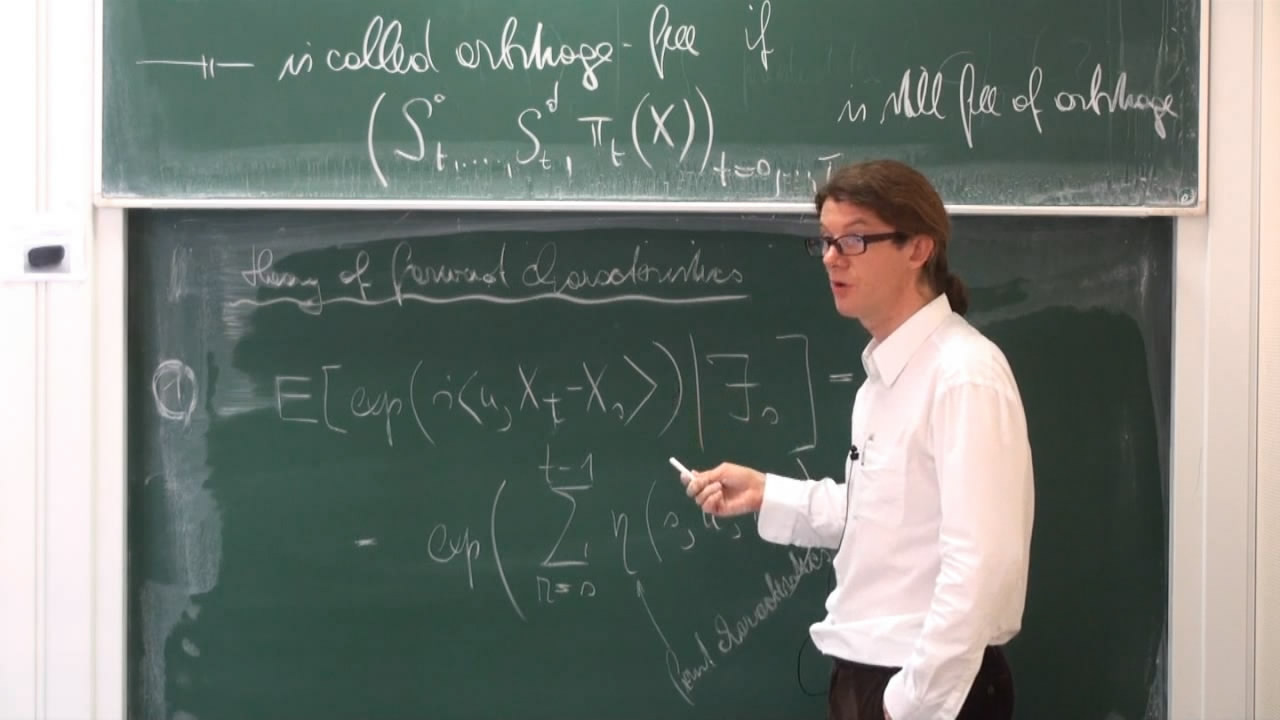# Discrete Time Theory of Term Structure Models

Курс Хит
Предмет:
Лектор:Stochastically evolving term structures of prices appear in interest rate theory, credit risk theory and option pricing. We present the discrete time version of this theory, which can also be regarded as useful discretization theory of a continuous time theory.

Lecture 1: No arbitrage in discrete time for multi-period models.

Lecture 2: Term structures of interest rates in discrete time, Term structures of option prices in discrete time.

Lecture 3: Dynamics of term structures in discrete time, finite dimensional realizations.

Prerequisities: Probability theory in discrete time and some stochastic analysis

Страница лекции на сайте лаборатории им.П.Л.Чебышева.

3